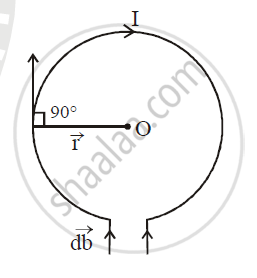Share

# Obtain an Expression for Magnetic Flux Density B at the Centre of a Circular Coil Of Radius R, Having N Turns and Carrying a Current I - ISC (Science) Class 12 - Physics (Theory)

#### Question

Obtain an expression for magnetic flux density B at the centre of a circular coil of radius R, having N turns and carrying a current I

#### Solution

Magnetic flux density B at the centre of a circular coil of radius r having N turns and carrying a current I.Consider a circular coil of radius r and carrying the current I in the direction as shown in the figure. Suppose the entire circular coil is divided into a large number of current elements, each of length dl. According to Biot-savart law, the magnetic field vec(dB) at the centre O of the coil due to current element Ivec(dl) is given by

vec(dB) = mu_0/(4pi) (I(vec(dl) xx vecr))/r^3

The magnitude of vec(db) at the centre O is

dB = mu_0/(4pi) (Idl xx r sin theta)/(r^3)

db  = mu_0/(4pi) (I dl sin theta)/r^2

.B = int dB

= int mu_0/(4pi) (i dl sin theta)/r^2

theta= 90^@ ∴ sin 90^@ = 1

:. B = mu_o/(4pi) I/r^2 int dldl

intdl= total length of the  coil = 2pir

B = mu_o/(4pi) I/r^2 (2pir)

B =(mu_oI)/(2r)

If the coil N turns

:. B = (mu_oNI)/(2r)

Is there an error in this question or solution?

#### APPEARS IN

2014-2015 (March) (with solutions)
Question 6.1 | 3.00 marks

#### Video TutorialsVIEW ALL 

Solution Obtain an Expression for Magnetic Flux Density B at the Centre of a Circular Coil Of Radius R, Having N Turns and Carrying a Current I Concept: Magnetic Force.
S